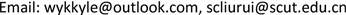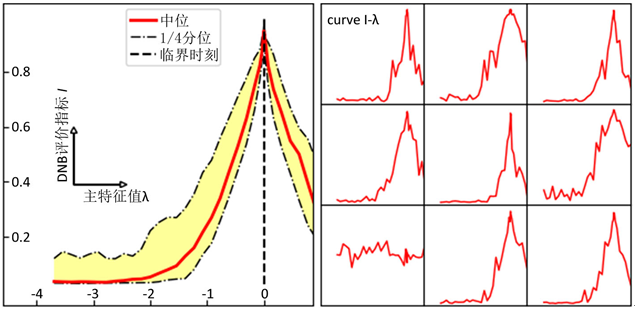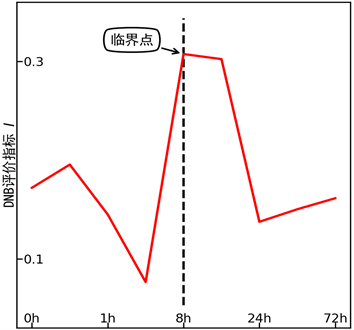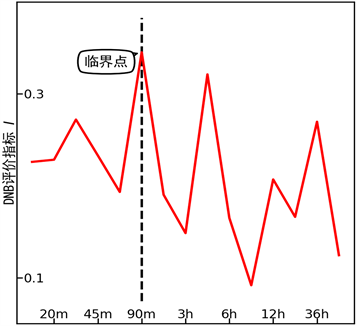﻿ 采用PageRank算法探测生物过程中的临界点 Identifying Critical Transition with PageRank Algorithm in a Biological Process

Vol. 09  No. 02 ( 2020 ), Article ID: 34229 , 7 pages
10.12677/AAM.2020.92026

Identifying Critical Transition with PageRank Algorithm in a Biological Process

Yangkai Wang, Rui Liu

School of Mathematics, South China University of Technology, Guangzhou GuangdongReceived: Jan. 30th, 2020; accepted: Feb. 12th, 2020; published: Feb. 19th, 2020ABSTRACT

With the belief that high-throughput datasets hold all the necessary information we want, a problem of information retrieval confronts us. As PageRank algorithm achieves a great success in dealing with such a problem in the field of Internet, we adapt it for high-throughput datasets in combination with the theory of dynamical network biomarker, and try to identify a critical transition in the biological processes. Our adapted PageRank algorithm successfully identifies the designated critical points in data simulations and it also produces the same results with the earlier works when applied to experimental datasets.

Keywords:Pagerank Algorithm, Dynamical Network Biomarker (DNB), Critical Phenomena, High Throughput Gene Expression Profiling1. 引言

PageRank是一个受到广泛研究的算法，在不同应用情景下有着多样的具体形式 。基本地，PageRank算法就是求取如下迭代的平衡点：

$\pi ←\alpha {H}_{n}^{\text{T}}\pi +\alpha \left({d}^{\text{T}}\pi \right){v}_{n}+\left(1-\alpha \right){v}_{n},$ (1)

2. 方法

2.1. 从PCC矩阵提取邻接矩阵

$\sqrt{{r}^{2}\left(n-2\right)/\left(1-{r}^{2}\right)}~{t}_{n-2}$ (2)

${H}_{r}\left[i,j\right]=\left(|R\left[i,j\right]|-{T}_{c}\right)/\left(1-{T}_{c}\right)\ast {|R\left[i,j\right]|}^{n}$ (3)

2.2. 抑制网络中的背景结构

$\begin{array}{l}{H}_{m}\left[i,j\right]={H}_{r}\left[i,j\right]\left(1-S\left[i,j\right]\right)/\left(1+{\sum }_{i}S\left[i,j\right]\right)\\ {\pi }_{m}\left[i\right]={\pi }_{o}\left[i\right]\left(1+{\sum }_{i}S\left[i,j\right]\right)\end{array}$ (4)

2.3. 弥合悬挂节点的不连续性

2.4. DNB子网络中的PageRank值

$E=\left({P}_{G}+\left({P}_{I}-{P}_{o}\right)-\left({P}_{d}-{P}_{t}\right)\right)/\left(1-\alpha \right)$ (5)

${P}_{G}$ 是由个性化向量产生的PageRank值， ${P}_{I}$${P}_{o}$ 分别是通过边输入和输出的PageRank值， ${P}_{d}$${P}_{t}$ 分别是子网络中经由悬挂节点向全网逸散的PageRank值以及由全体悬挂节点逸散而来的PageRank值。对DNB子网络：由于内部紧密相关，所以 ${P}_{d}$ 是0；又由于DNB相对独立， ${P}_{I}$${P}_{o}$ 都小，理想情况下是0。 ${P}_{t}$ 实践中发现也不大，虽然 ${P}_{t}$ 使得E增大，对我们的算法有利。这样 $E\approx {P}_{G}/\left(1-\alpha \right)={\sum }_{i}^{\text{DNB}}{v}_{n}\left[i\right]$， 取遍DNB中所有节点。

2.5. 渐进PageRank方法

2.6. DNB评价指标

$I\stackrel{\text{def}}{=}{\sum }_{i\ne j}^{\text{DNB}}{H}_{r}\left[i,j\right]/\left(n\left(n-1\right)\right)$ (6)

3. 结果

3.1. 数值模拟

$x←\text{exp}\left(TD\left(\beta \right){T}^{-1}\right)x+\xi$ (7)

$x$ 是迭代变量，初值为全0； $\xi$ 是随机向量，各分量独立服从高斯分布，用于在迭代过程中引入随机性。在模拟数据集合中，我们的渐进PageRank算法成功定位了DNB并给出了临界预警信号。在图1中我们展示了DNB评价指标随主特征值 的变化；在绝大多数模拟数据中，DNB评价指标在 $\lambda$ 趋于0时趋近于1，成功地指示了临界点。Figure 1. DNB indication for simulations (left: a synthesis result, right: specific curves)

3.2. 实验数据Figure 2. DNB indication for the experiment of mouse lung injuryFigure 3. DNB indication for the experiment of HRG induced differentiation on MCF-7 human breast cancer cells

4. 讨论

Identifying Critical Transition with PageRank Algorithm in a Biological Process[J]. 应用数学进展, 2020, 09(02): 231-237. https://doi.org/10.12677/AAM.2020.92026

1. 1. Scheffer, M., Bascompte, J., Brock, W.A., et al. (2016) Early-Warning Signals for Critical Transitions. Nature, 461, 53-59. https://doi.org/10.1038/nature08227

2. 2. Chen, L., Liu, R., Liu, Z.P., et al. (2012) Detecting Early-Warning Signals for Sudden Deterioration of Complex Diseases by Dynamical Network Biomarkers. Scientific Reports, 2, Article No. 342. https://doi.org/10.1038/srep00342

3. 3. Liu, R., Wang, X., Aihara, K. and Chen, L. (2014) Early Diagnosis of Complex Diseases by Molecular Biomarkers, Network Biomarkers, and Dynamical Network Biomarkers. Medicinal Research Reviews, 34, 455-478. https://doi.org/10.1002/med.21293

4. 4. Chen, P., Li, Y., Liu, R. and Chen, L. (2017) Detecting the Tipping Points in a Three-State Model of Complex Diseases by Temporal Differential Networks. Journal of Translational Medicine, 15, 217. https://doi.org/10.1186/s12967-017-1320-7

5. 5. Langville, A.N. and Meyer, C.D. (2011) Google’s PageRank and Beyond: The Science of Search Engine Rankings. Princeton University Press, Princeton.

6. 6. Szklarczyk, D., Morris, J.H., Cook, H., et al. (2017) The STRING Database in 2017: QUALITY-Controlled Protein-Protein Association Net-works, Made Broadly Accessible. Nucleic Acids Research, 45, D362-D368. https://doi.org/10.1093/nar/gkw937

7. 7. Wang, X., Tao, T., Sun, J., et al. (2010) DirichletRank: Ranking Web Pages Against Link Spams.

8. 8. Bianchini, M., Gori, M. and Scarselli, F. (2005) Inside PageRank. ACM Transactions on Internet Technology, 5, 92-128. https://doi.org/10.1145/1052934.1052938

9. 9. Becchetti, L. and Castillo, C. (2006) The Distribution of PageRank Follows a Power-Law Only for Particular Values of the Damping Factor. Pro-ceedings of the 15th International Conference on World Wide Web, Edinburgh, 23-26 May 2006, 941-942. https://doi.org/10.1145/1135777.1135955

10. 10. Sciuto, A.M., Phillips, C.S., Orzolek, L.D., et al. (2005) Genomic Analysis of Murine Pulmonary Tissue Following Carbonyl Chloride Inhalation. Chemical Research in Toxicology, 18, 1654-1660. https://doi.org/10.1021/tx050126f

11. 11. Saeki, Y., Endo, T., Ide, K., et al. (2009) Ligand-Specific Se-quential Regulation of Transcription Factors for Differentiation of MCF-7 Cells. BMC Genomics, 10, 545. https://doi.org/10.1186/1471-2164-10-545

12. 12. Nagashima, T., Shimodaira, H., Ide, K., et al. (2006) Quantitative Transcriptional Control of ErbB Receptor Signaling Undergoes Graded to Biphasic Response for Cell Differentiation. Journal of Biological Chemistry, 282, 4045-4056. https://doi.org/10.1074/jbc.M608653200